Question

Find the transfer function G(s)=Vo(s)/Vi(s) for the electrical network shown in the figure below. Express the...

Find the transfer function G(s)=Vo(s)/Vi(s) for the electrical network shown in the figure below. Express the transfer function G(s) as a ratio of polynomials.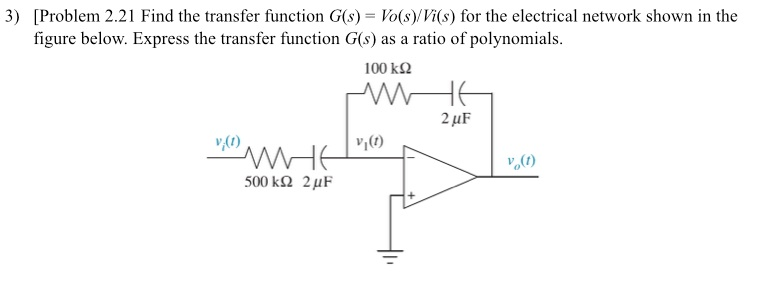So

Earn Coins

Coins can be redeemed for fabulous gifts.

Similar Homework Help Questions
• Find the transfer function G(s)=Vo(s)/Vi(s) for the electrical network shown in the figure below. Express the...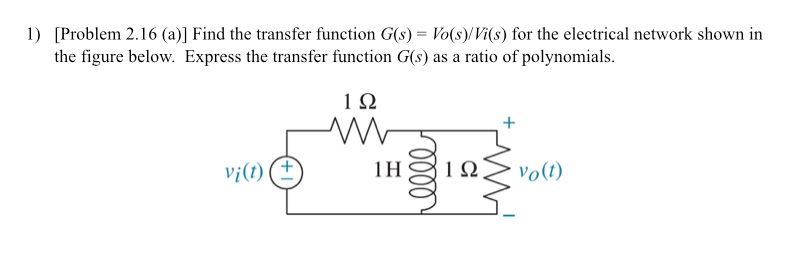Find the transfer function G(s)=Vo(s)/Vi(s) for the electrical network shown in the figure below. Express the transfer function G(s) as a ratio of polynomials.

• (i) Find the transfer function G(s) = Vo(s)/Vi(s) of this system using electrical impedances. Express the...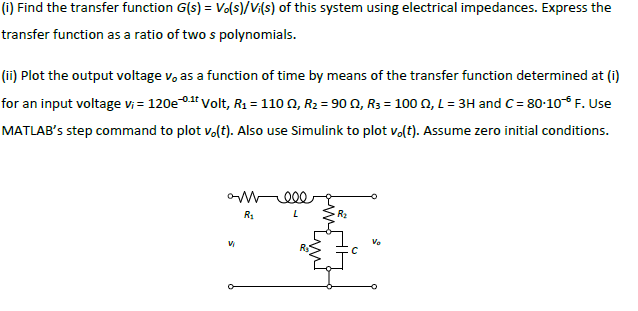(i) Find the transfer function G(s) = Vo(s)/Vi(s) of this system using electrical impedances. Express the transfer function as a ratio of two s polynomials. (ii) Plot the output voltage v, as a function of time by means of the transfer function determined at (i) for an input voltage vi= 120e0.18 Volt, R2 = 110 9, R2 = 900, R3 = 100 0, L = 3H and C= 80-106 F. Use MATLAB's step command to plot volt). Also use Simulink...

• Find the transfer function G(s) = V.(s)/Vi(s) for the network shown below. For full credit, make...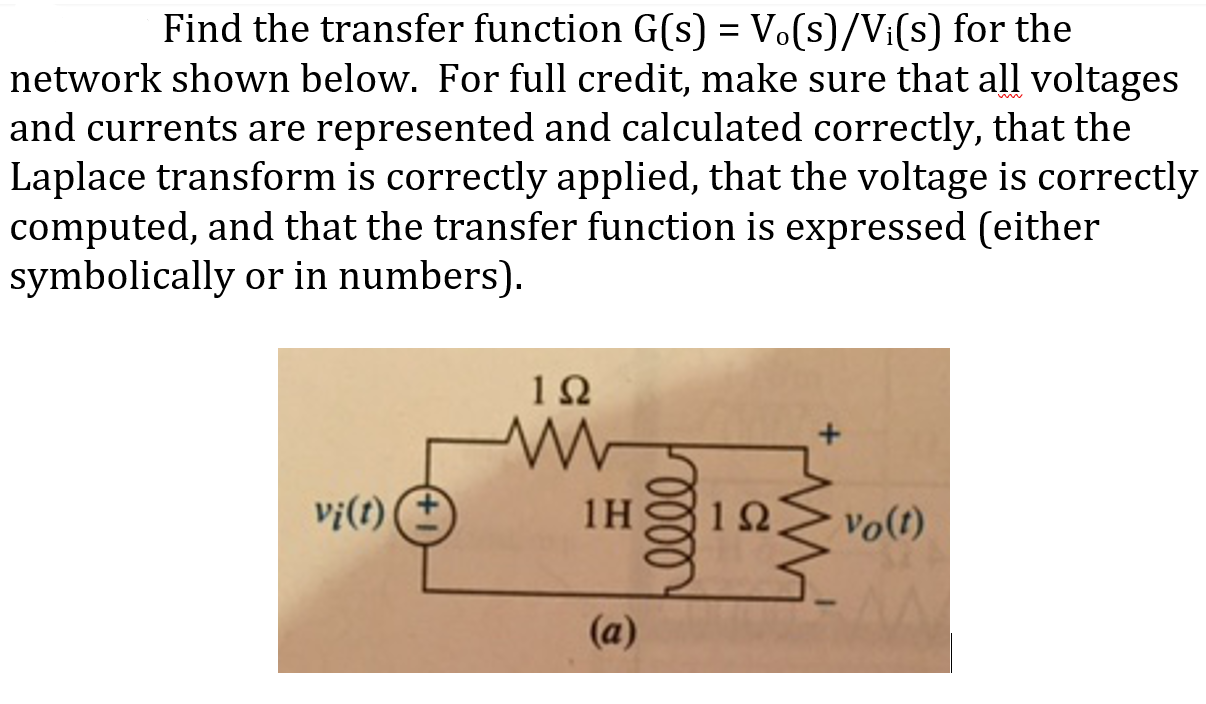Find the transfer function G(s) = V.(s)/Vi(s) for the network shown below. For full credit, make sure that all voltages and currents are represented and calculated correctly, that the Laplace transform is correctly applied, that the voltage is correctly computed, and that the transfer function is expressed (either symbolically or in numbers). 192 vi(t) 1Η 112 vo(t) (a)

• The transfer function Vo(s)/Vi(s) of the electrical system described in figure shown below is: * (3...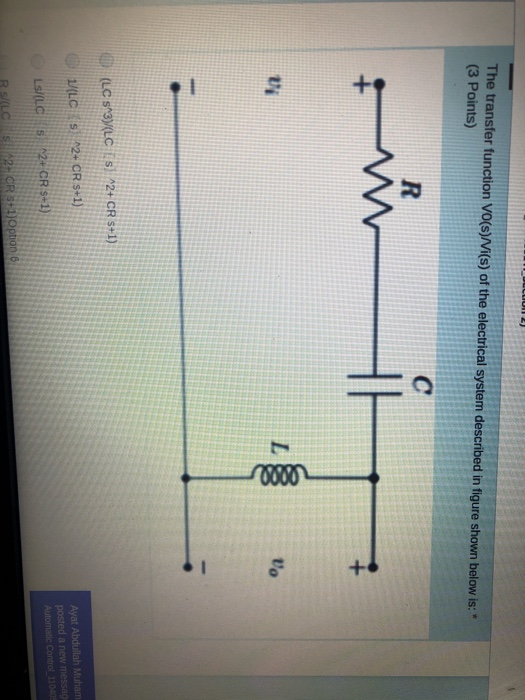The transfer function Vo(s)/Vi(s) of the electrical system described in figure shown below is: * (3 Points) R w + U L 10000 Vo (LCS"3(LC S) 12+ CR 5+1) 1/(LC s 2+ CR S+1) Ayat Abdullah Muham posted a new messag Automatic Control 110005 Ls/(C 2+ CR 5+1) 2. CR 5+1)Option 6

• The transfer function Vo(s)/Vi(s) of the electrical system described in figure shown below is. (3 Points...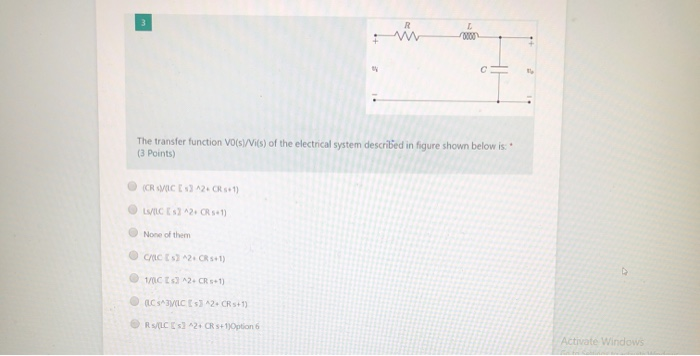The transfer function Vo(s)/Vi(s) of the electrical system described in figure shown below is. (3 Points (CRC) 12.1) LSIC 2.5+1) None of them CHCESZ 2CR5+1) 1/CE 2. CR 5+1) CSAVICE 2. CR3+1) R/LCES2 CRS+1)Option 6 Activate Windows

• Find the ratio Vo(s)/Vi(s) for the electrical network shown in the figure below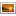Q1.png 1. Find the ratio Vds)/(s) for the electrical network shown in Fig. 5 1,6) R. V(+) C2 V. (D) b) For the transfer function in 5b, find the time response if m=1, B=3 and K=2 to an input Viſt)= 5u(t), where u(t) is unit step function

• Q1. For the filter circuit shown below, (5 marks) Vo(s) a) Find the transfer function, G(s)...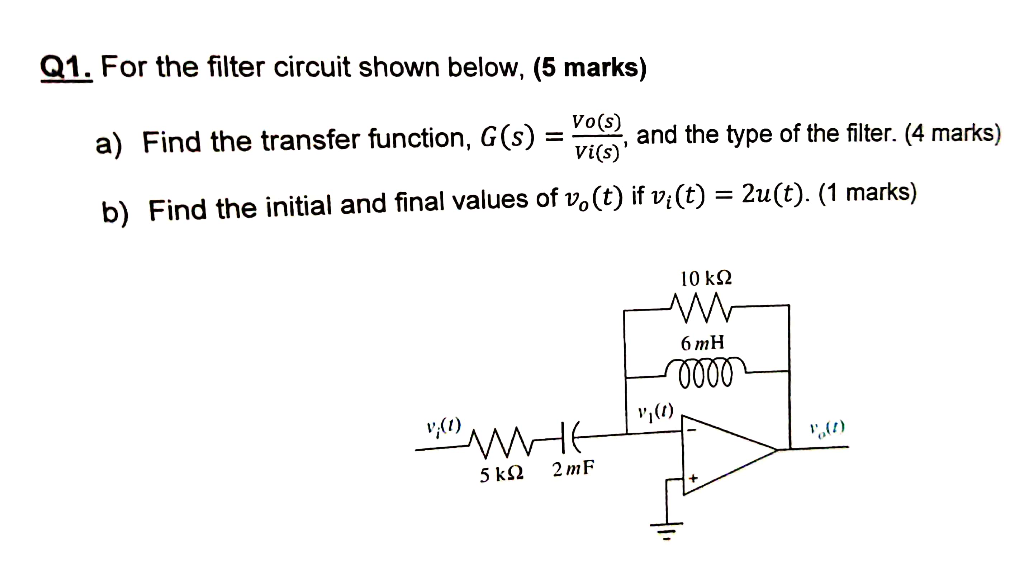Q1. For the filter circuit shown below, (5 marks) Vo(s) a) Find the transfer function, G(s) and the type of the filter. (4 marks) Vi(s)' b) Find the initial and final values of vo(t) if vi(t) = 2u(t). (1 marks) 10 k12 w 6 тн 0000 v;(1) 5 k92 2 mF

• Fall 2017 Find the transfer function, G(s) X.(s)/F(s), for the translational mechanical network shown in Figure...Fall 2017 Find the transfer function, G(s) X.(s)/F(s), for the translational mechanical network shown in Figure P1 1. X, t) 2 N 2Ka IN-5Im

• For the circuit shown below, a) Determine the transfer function vo/vi b) Plot lVolvil versus ω...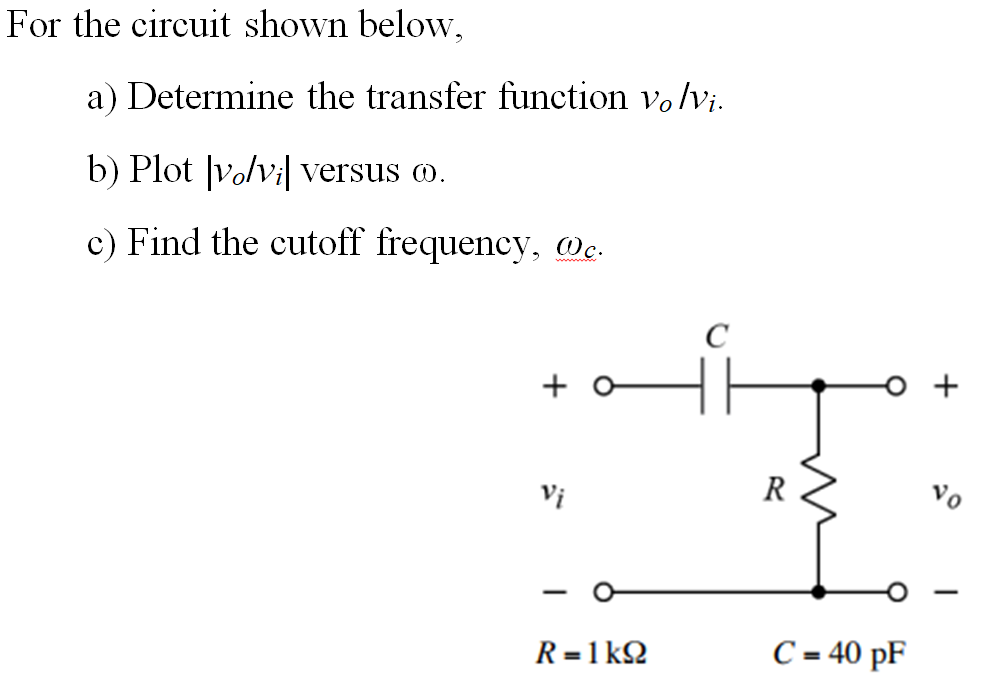For the circuit shown below, a) Determine the transfer function vo/vi b) Plot lVolvil versus ω c) Find the cutoff frequency, n. Vi C=40pF

• Problem 4 17. Find the transfer function, G(s) = VL(s)/V(s), for each network shown in Figure...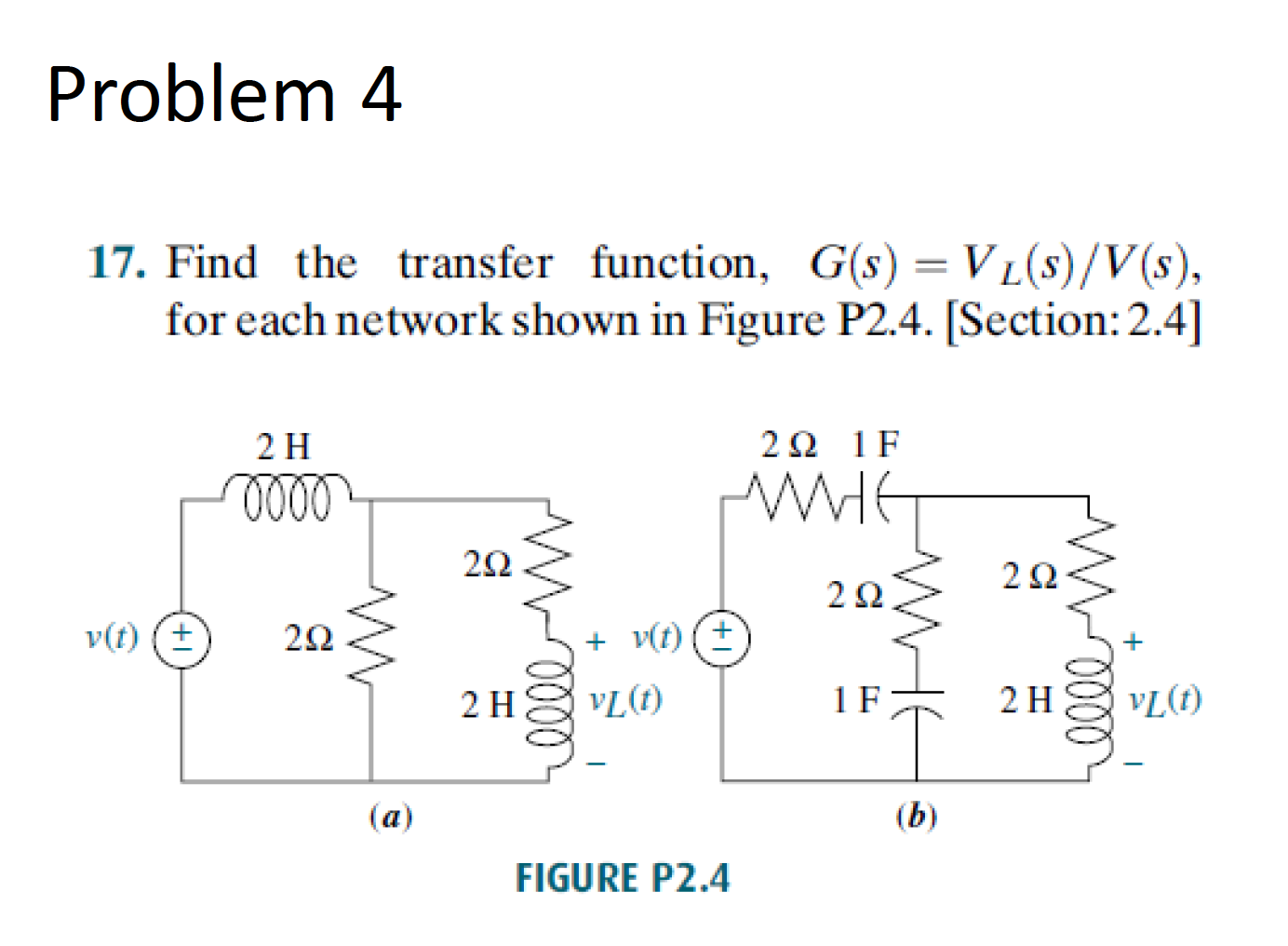Problem 4 17. Find the transfer function, G(s) = VL(s)/V(s), for each network shown in Figure P2.4. [Section: 2.4] 2 H 22 1F -1000 v(t) 203 v(t) (+) 2hvLT 21 vL (1) - (a) FIGURE P2.4